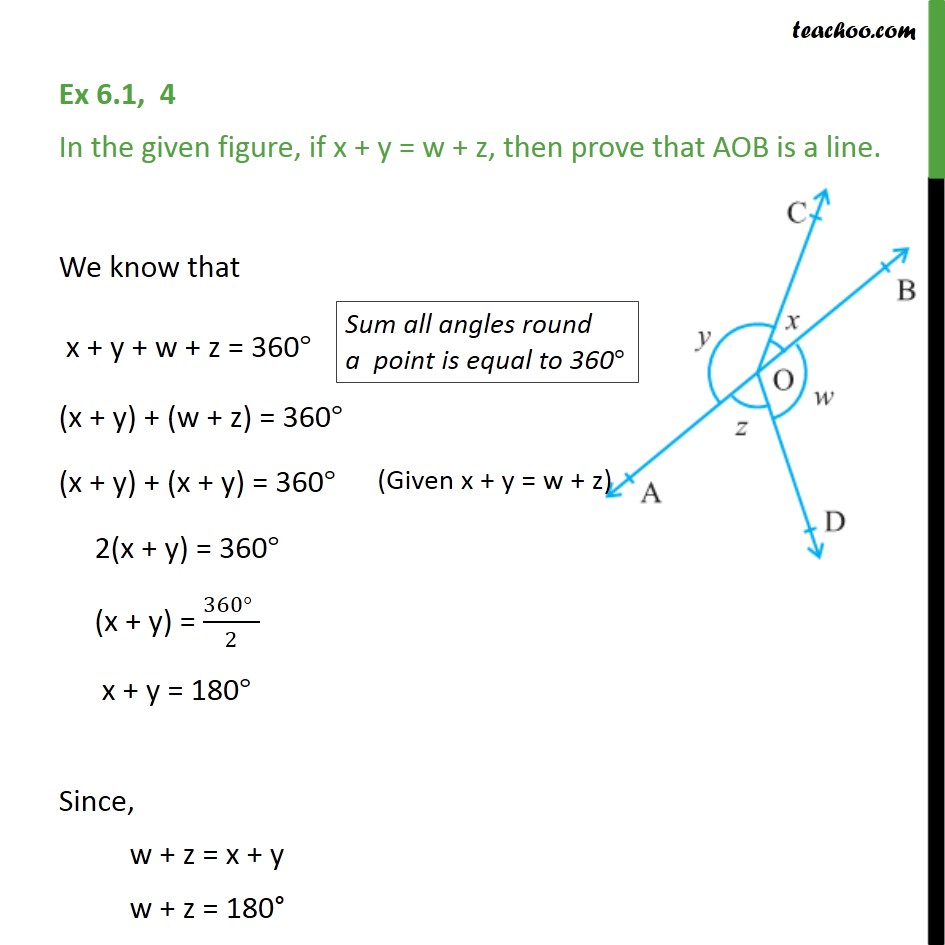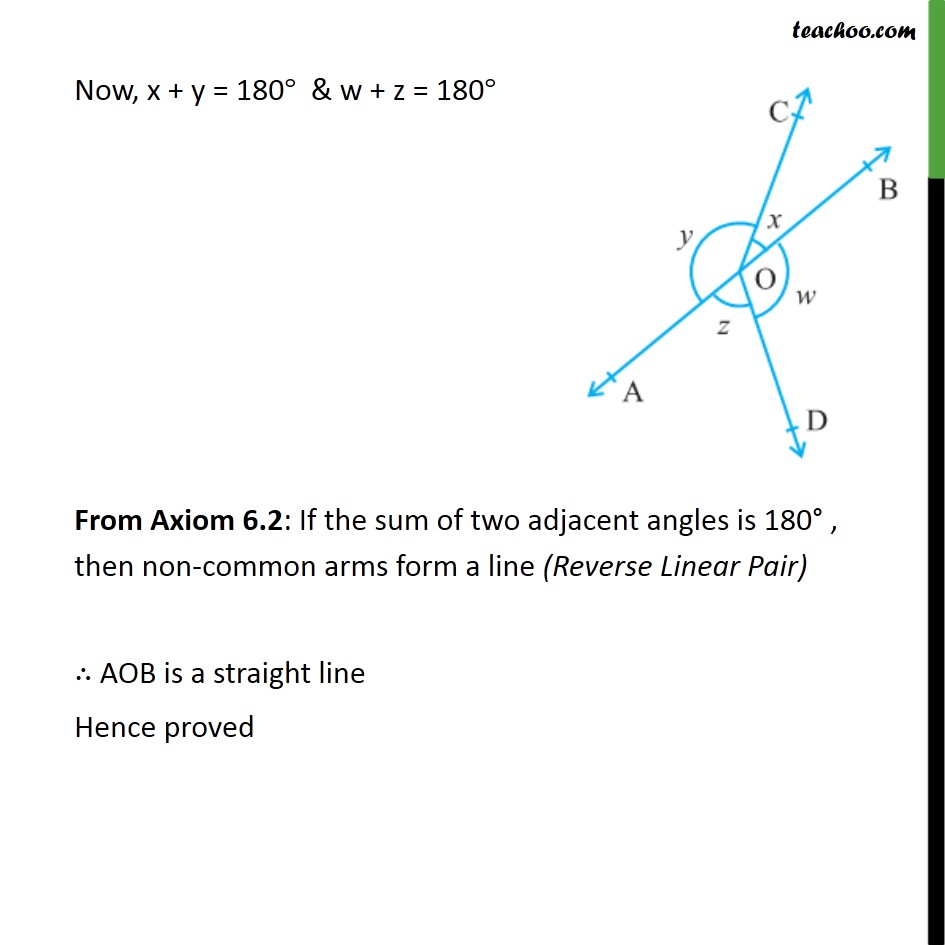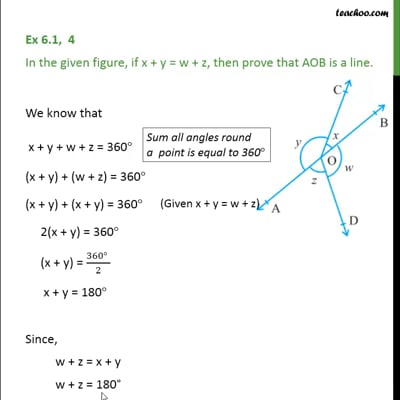Ex 6.1

Chapter 6 Class 9 Lines and Angles
Serial order wiseThis video is only available for Teachoo black users

Solve all your doubts with Teachoo Black (new monthly pack available now!)

### Transcript

Ex 6.1, 4 In the given figure, if x + y = w + z, then prove that AOB is a line. We know that x + y + w + z = 360° (x + y) + (w + z) = 360° (x + y) + (x + y) = 360° 2(x + y) = 360° (x + y) = (360°" " )/2 x + y = 180° Since, w + z = x + y w + z = 180° Now, x + y = 180° & w + z = 180° From Axiom 6.2: If the sum of two adjacent angles is 180° , then non-common arms form a line (Reverse Linear Pair) ∴ AOB is a straight line Hence proved